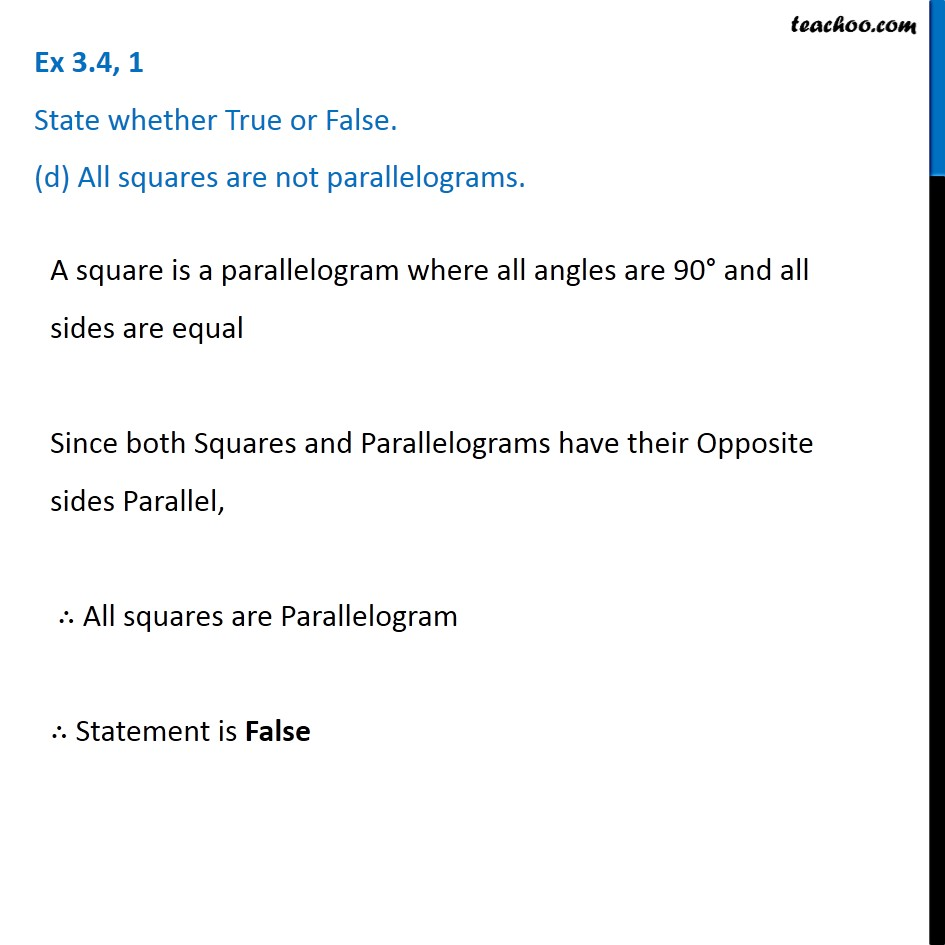1. Chapter 3 Class 8 Understanding Quadrilaterals
2. Serial order wise
3. Ex 3.4

Transcript

Ex 3.4, 1 State whether True or False. (d) All squares are not parallelograms. A square is a parallelogram where all angles are 90° and all sides are equal Since both Squares and Parallelograms have their Opposite sides Parallel, ∴ All squares are Parallelogram ∴ Statement is False